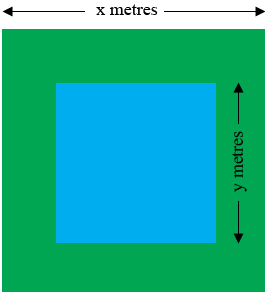SEARCH HOMEMath Central Quandaries & QueriesQuestion from Jin: I have a large square pond set inside a square garden: both the pond and the garden have sides which are a whole number of metres, and outside the pond, the garden is grassed over. The area covered by grass is 188 square metres. Find the area of the pond. (5 marks)Hi Jin,

I can help get you started.

Here is my sketch of the garden and pond. The garden measures $x$ metres on a side and the pond measures $y$ metres on a side. $x$ and $y$ are both whole numbers.The area of the grass is the area of the $x$ by $x$ square minus the area of the surface of the pond. Thus

$x^2 - y^2 = 188$

Can you complete the problem now?

Write back if you need more assistance,
PennyMath Central is supported by the University of Regina and The Pacific Institute for the Mathematical Sciences.